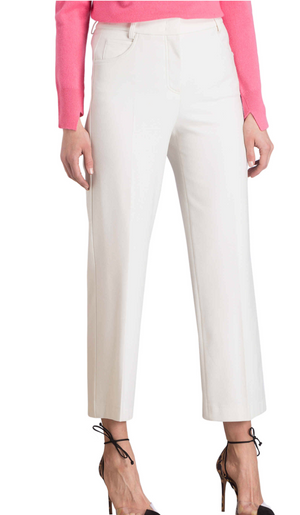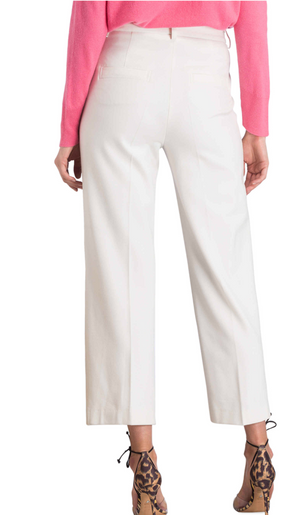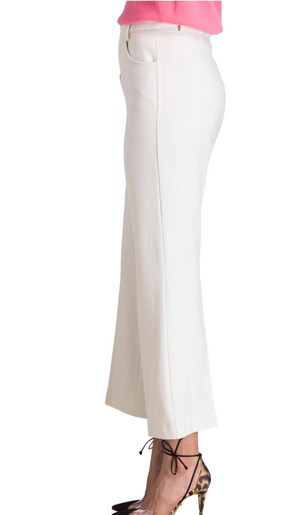Raffaello Rossi

# Drona

Regular price \$295 now \$147.50 Unit price  per

Shipping calculated at checkout.
• 63% polyester 33% viscose 4% elastane
• Hand wash only
• Ankle length
• High rise
• Gaucho style
• Fits true to size

Size Guide

• 32 = 2 = XXS
• 34 = 4 = XS
• 36 = 6 = S
• 38 = 8 = M
• 40 = 10 = M
• 42 = 12 = L
• 44 = 14 = L
• 46 = 16 =XL